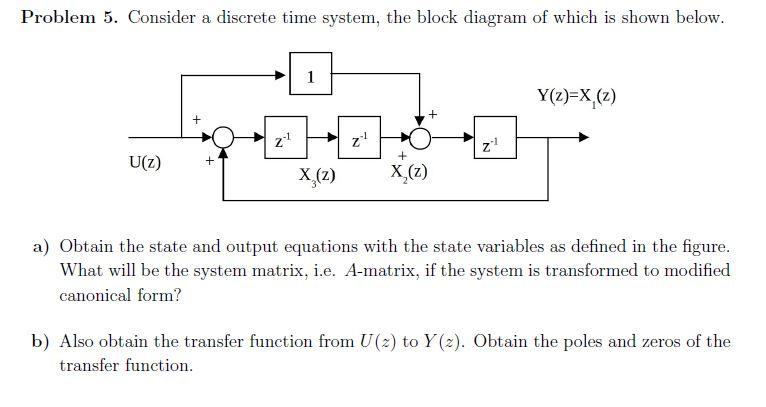# Question Solved1 AnswerProblem 5. Consider a discrete time system, the block diagram of which is shown below. a) Obtain the state and output equations with the state variables as defined in the figure. What will be the system matrix, i.e. A-matrix, if the system is transformed to modified canonical form? b) Also obtain the transfer function from \( U(z) \) to \( Y(z) \). Obtain Problem 5. Consider a discrete time system, the block diagram of which is shown below. a) Obtain the state and output equations with the state variables as defined in the figure. What will be the system matrix, i.e. A-matrix, if the system is transformed to modified canonical form? b) Also obtain the transfer function from \( U(z) \) to \( Y(z) \). Obtain the poles and zeros of the transfer function.QVJIV5 The Asker · Electrical EngineeringTranscribed Image Text: Problem 5. Consider a discrete time system, the block diagram of which is shown below. a) Obtain the state and output equations with the state variables as defined in the figure. What will be the system matrix, i.e. A-matrix, if the system is transformed to modified canonical form? b) Also obtain the transfer function from \( U(z) \) to \( Y(z) \). Obtain the poles and zeros of the transfer function.
More
Transcribed Image Text: Problem 5. Consider a discrete time system, the block diagram of which is shown below. a) Obtain the state and output equations with the state variables as defined in the figure. What will be the system matrix, i.e. A-matrix, if the system is transformed to modified canonical form? b) Also obtain the transfer function from \( U(z) \) to \( Y(z) \). Obtain the poles and zeros of the transfer function.